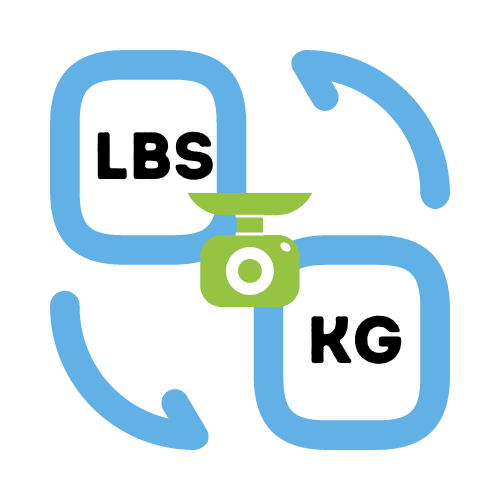# Convert Pounds (lbs) to Kilograms (kg)

## Simple conversion of pounds to kilograms.

• Created by Emily Rodriguez
• Reviewed by Ramesh Agarwal
• Last updated 26th October 2023
Enter a number.

## How to use the Weight Calculator

Simply enter your weight in pounds and then press the "Calculate" button. The calculator will convert the pounds value to kg instantly. If you want to convert kg to pounds then we have a weight calculator for that too.

## How do you convert pounds to kg?

Each pound is equal to 0.45 kilogram (kg). From the opposite perspective, 1kg has a weight of 2.205 pounds (lbs). To calculate the weight in pounds or kilograms we can simply multiple or divide by 2.205, depending on which value we start with.

Here is an example conversion for pounds (lbs) to kilograms (kg):
160 pounds in kg can be calculated as `160 / 2.205 = 72.56kg`

## Where did the pound weight come from?

Weight is typically measured in pounds in the United States and some other countries because it is part of the imperial system of units, which is used in those countries.

The imperial system is a system of measurement that was originally developed in the United Kingdom and is still used in a number of countries around the world, including the United States. In the imperial system, weight is typically measured in pounds (lb) and ounces (oz).

On the other hand, the metric system is a system of measurement that is based on the International System of Units (SI) and is used in most other countries around the world. In the metric system, weight is typically measured in kilograms (kg) and grams (g).

The kilogram is the base unit of mass in the metric system, and it is defined as the mass of a specific reference object, called the International Prototype Kilogram, which is kept at the International Bureau of Weights and Measures in France. The gram is a unit of mass that is equal to 1/1000 of a kilogram.

## What is the pounds to kilograms conversion formula?

The formula for converting pounds to kilograms is:
`kg = lb / 2.205`

## How do I convert kilograms to pounds?

To convert kilograms to pounds, you can use the following formula:
`lb = kg * 2.205`

## Pounds to Kilograms Conversion Table

This table shows values in Pounds (lb) and their equivalent values in Kilograms (kg).

Pounds (lb) Kilograms (kg)
1 0.45
2 0.91
3 1.36
4 1.81
5 2.27
6 2.72
7 3.18
8 3.63
9 4.08
10 4.54
15 6.8
20 9.07
25 11.34
30 13.61
35 15.87
40 18.14
45 20.41
50 22.68